Full Text:<2593>

Summary:<2031>

CLC number: U448.25

On-line Access: 2014-10-08

Revision Accepted: 2014-09-17

Crosschecked: 2014-09-25

Cited: 3

Clicked: 6853

Citations:  Bibtex RefMan EndNote GB/T7714

 Journal of Zhejiang University SCIENCE A 2014 Vol.15 No.10 P.798-812 http://doi.org/10.1631/jzus.A1400194A simulation study on the optimal control of buffeting displacement for the Sutong Bidge with multiple tuned mass dampers*

 Author(s):  Hao Wang, Tian-you Tao, Huai-yu Cheng, Ai-qun Li Affiliation(s):  . Key Laboratory of Concrete and Prestressed Concrete Structure of Ministry of Education, Southeast University, Nanjing 210096, China Corresponding email(s):   wanghao1980@seu.edu.cn Key Words:  Long-span cable-stayed bridge, Buffeting response, Vibration control, Multiple tuned mass dampers (MTMDs), Control efficiency Share this article to： More <<< Previous Article|Next Article >>>

Hao Wang, Tian-you Tao, Huai-yu Cheng, Ai-qun Li. A simulation study on the optimal control of buffeting displacement for the Sutong Bridge with multiple tuned mass dampers[J]. Journal of Zhejiang University Science A, 2014, 15(10): 798-812.

@article{title="A simulation study on the optimal control of buffeting displacement for the Sutong Bridge with multiple tuned mass dampers",
author="Hao Wang, Tian-you Tao, Huai-yu Cheng, Ai-qun Li",
journal="Journal of Zhejiang University Science A",
volume="15",
number="10",
pages="798-812",
year="2014",
publisher="Zhejiang University Press & Springer",
doi="10.1631/jzus.A1400194"
}

%0 Journal Article
%T A simulation study on the optimal control of buffeting displacement for the Sutong Bridge with multiple tuned mass dampers
%A Hao Wang
%A Tian-you Tao
%A Huai-yu Cheng
%A Ai-qun Li
%J Journal of Zhejiang University SCIENCE A
%V 15
%N 10
%P 798-812
%@ 1673-565X
%D 2014
%I Zhejiang University Press & Springer
%DOI 10.1631/jzus.A1400194

TY - JOUR
T1 - A simulation study on the optimal control of buffeting displacement for the Sutong Bridge with multiple tuned mass dampers
A1 - Hao Wang
A1 - Tian-you Tao
A1 - Huai-yu Cheng
A1 - Ai-qun Li
J0 - Journal of Zhejiang University Science A
VL - 15
IS - 10
SP - 798
EP - 812
%@ 1673-565X
Y1 - 2014
PB - Zhejiang University Press & Springer
ER -
DOI - 10.1631/jzus.A1400194

Abstract:
The buffeting of long-span cable-supported bridges under strong winds is one of the key issues in bridge wind engineering. In order to study the effectiveness of the multiple tuned mass dampers (MTMDs) in buffeting control of long-span bridges, the Sutong Cable-stayed Bridge (SCB) with a main span of 1088 m in China is taken as an example in this paper. The spatial finite element model of the SCB is established and the modal analysis is conducted based on ANSYS. After the 3D turbulence wind field of the SCB is simulated using the measured wind parameters, the time-domain buffeting analysis on the SCB is conducted with the aerodynamic self-excited forces included. According to the dynamic characteristics and the time-domain buffeting analysis results of the SCB, the parameter sensitivity analysis on buffeting vibration control with MTMD is conducted in ANSYS. The optimum parameters are then obtained with the construction difficulty and economic factors considered. Results show that the control efficiency is sensitive to the number of the TMD, mass ratio, frequency band-width ratio, and damping ratio. Both the vertical and the lateral vibrations can be effectively controlled when proper design parameters of a MTMD system are used. In addition, the control effect on lateral vibration is better than that on vertical vibration. Results obtained in this study can provide references for anti-wind design and buffeting control of long-span cable-stayed bridges.

## 1.  Introduction

With the development of transportation in China, a large number of bridges have been constructed over rivers, seas, and canyons. However, with the increase of the bridge span, the bridges are becoming more and more sensitive to the actions of wind loads, which is usually due to the decrease of structural stiffness (Simiu and Scanlan, ). As one of the most common vibrations in bridges, turbulence-induced buffeting will decrease the driving comfort and cause fatigue failure. Therefore, buffeting has a great impact on the performance of long-span bridges. A lot of researches on buffeting analysis have been conducted. For instance, Xu et al. () presented a new algorithm for buffeting analysis of long-span bridges, featuring a complete finite element approach and a pseudo-excitation method. Chen et al. () conducted an investigation on the influence factors of the buffeting response of bridges with a time-domain procedure, taking into account the self-excited forces, aerodynamic admittance functions and the coherence of buffeting forces. Chen and Kareem () proposed a framework based on a complex modal decomposition technique for predicting the coupled buffeting responses of bridges in both time and frequency domains, with an example to illustrate the proposed scheme and its effectiveness. Based on the wind data from the structural health monitoring system (SHMS), Wang et al. () conducted a comparative study on the buffeting performance of the Sutong Cable-stayed Bridge (SCB) based on the design and measured spectrum. All of the above researches demonstrate the significance of the buffeting analysis for long-span bridges.

With the increase of the bridge span, wind-induced buffeting plays a dominant role in design, especially for those bridges located in the regions where typhoons or hurricanes often occur. To increase the structural stability and safety, effective control measures should be adopted to reduce wind-induced buffeting vibrations in long-span bridges. All the theoretical analyses, experimental researches, and practical uses have proven that the tuned mass damper (TMD) is an effective device for controlling structural vibrations (Gu et al., ). TMD is a classical engineering device with a mass, a spring, and a damper attached to attenuate undesirable vibrations of engineering structures. The optimum parameters’ analyses of the TMD for vibration control of systems under external forces have been effectively conducted (Den Hartog, ; Fujino and Abe, ; Rüdinger, ; Marano et al., ; ; Hoang et al., ; Marano and Greco, ). However, it is well known that the performance of a TMD is sensitive to the variation of frequency ratios between the TMD and structures. Efforts to prevent this disadvantage have been made, since it was realized, and consequently the problem can be solved with multiple tuned mass dampers (MTMDs). With several TMDs attached to the main structure, MTMDs have distributed natural frequencies around the controlled mode. A wide frequency band width makes it more robust and less sensitive to the variation of the frequency ratio than using TMD (Igusa and Xu, ). Moreover, the whipping effect gives MTMD the characteristics of extensive energy dissipation and high consumption rates, so relevant researches on optimum parameters’ design or its application to practical engineering have been widely conducted. For example, Abe and Fujino () analytically studied the modal characteristics and efficiencies of MTMD and proposed an explicit formula to estimate its effectiveness. Li () searched for optimum parameters of MTMD to determine the influence of important parameters on their effectiveness and robustness based on the displacement dynamic magnification factor and the acceleration dynamic magnification factor. Following preliminary investigation of MTMD, Kareem and Kline () investigated its effectiveness under narrow or wide banded excitations. Nguyen et al. () conducted experimental and numerical studies on the performance of a custom-made distributed multiple viscoelastic TMD system in suppressing the vibration level on an actual office floor subjected to various types of excitations. However, most of the researches on structural vibration control using the empirical spectrum formula to simulate the excitation, inevitably would deviate from real conditions and cause errors in analytical results.

In this paper, the SCB with a main span of 1088 m is identified as the research object. To record the wind surroundings and structural conditions of the SCB in real-time, a SHMS is installed on the bridge. The 3D turbulence wind field of the SCB is simulated using the measured wind parameters. Based on the dynamic characteristics and the buffeting analysis results of the SCB, the parameter sensitivity analysis on buffeting vibration control with MTMD is conducted. The optimum parameters are then acquired based on the first-order optimization method (Wang et al., ). In the view of both time-domain and frequency-domain, the vibrations of the vertical and lateral directions are well attenuated and the negative energy is effectively dissipated with the system of MTMD. The analytical results show the effectiveness of MTMD in buffeting control of long-span cable-stayed bridges, and therefore can provide references for anti-wind design of long-span bridges with similar features.

## 2.  Engineering background

### 2.1.  Description of the Sutong Cable-stayed Bridge

Located in the eastern part of the Jiangsu province in China, the SCB is near the entrance of the Yangtze River to the Yellow Sea (Fig. 1). As a super-large bridge project, the SCB with a main span of 1088 m was constructed with the highest construction standards and the most advanced techniques. When it was opened to traffic in May, 2008, the SCB was a cable-stayed bridge with the longest main span in the entire world.

SCB is a double-tower cable-stayed bridge with two cable planes (Fig. 2a). The total length of the girder is 2088 m with two auxiliary piers settled in each side span. A streamline flat steel-box-girder is employed as the bridge deck and the wind mouth is adopted to improve structural aerodynamic performance (Fig. 2b). Parallel wire cables are adopted for the structural suspension system, and the typical distance between each cable on the deck is 16 m.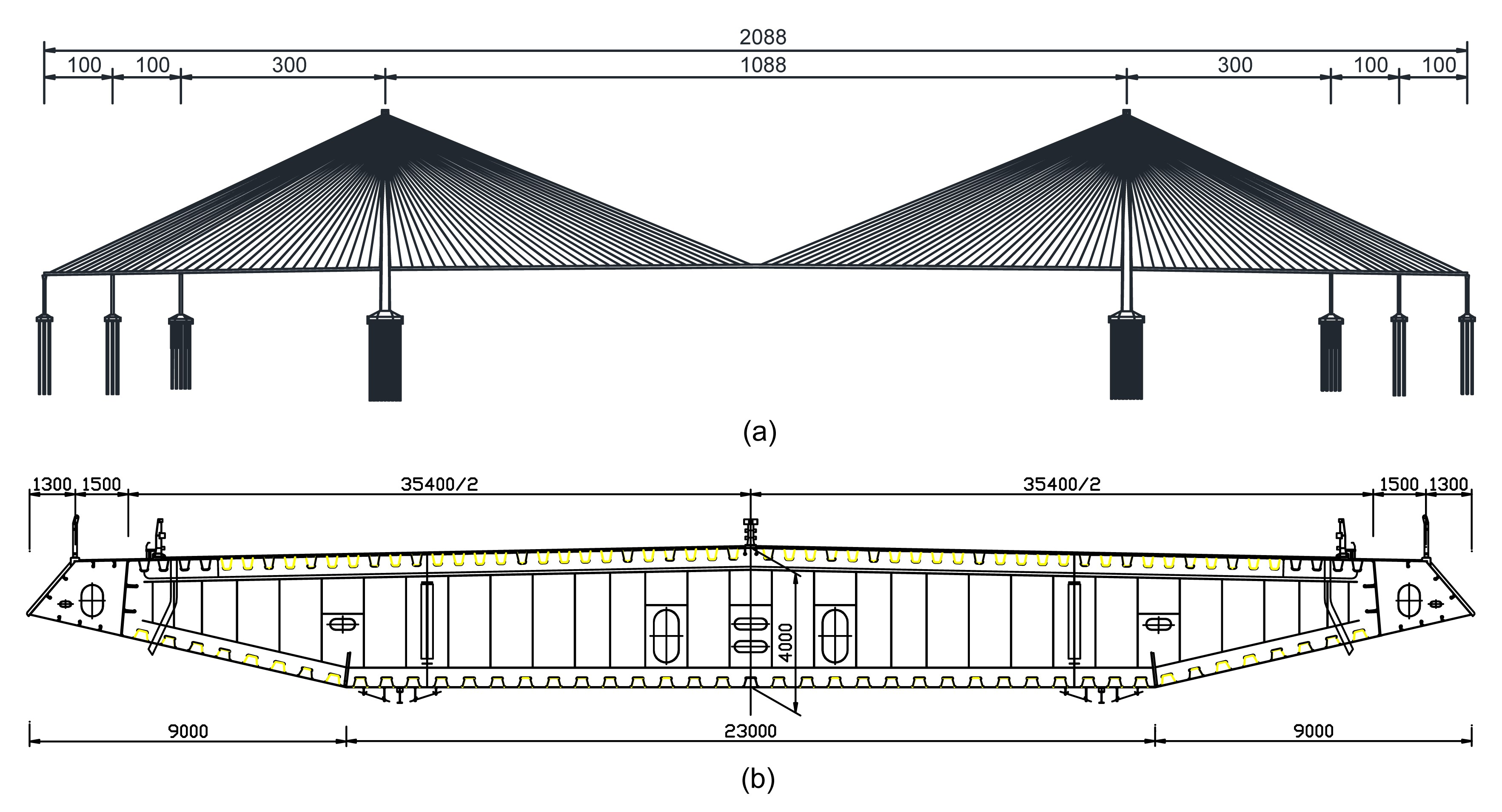Fig.2
Configurations of the Sutong Cable-stayed Bridge
(a) Elevation (unit: m); (b) Cross-section of the steel-box-girder (unit: mm)

### 2.2.  Climate at the bridge site

In the southeastern region of the Asian continent, there exists a humid subtropical monsoon climate, primarily due to the sea-land thermal differences in this region. The monsoon circulation usually leads to an obvious seasonal climate (Foster and Chan, ; Chan and Lee, ), especially a typhoon climate from the eastern ocean in summer and the north wind from Siberia in the northwest of China in winter. Therefore, as a long-span flexible structure, the anti-wind performance of the SCB during typhoons and strong winds requires special attention. As a typical example, Fig. 3 shows the recorded wind samples of Typhoon Haikui which passed through the SCB site in August, 2012 (Wang et al., ).Fig.3
Typhoon Haikui recorded by SHMS of the SCB (2012-08-08T00:00–2012-08-08T20:00)
(a) Wind speed; (b) Wind direction

## 3.  Simulation of a 3D fluctuating wind field

Owing to the large span and the complex structural system, wind-induced motions of the SCB are relatively complicated and its behavior shows strong nonlinearity caused by geometrical and aerodynamic effects. To compute the structural buffeting responses in time-domain, a 3D wind field is essential to be used as input components (Simiu and Scalan, ; Wang et al., ).

### 3.1.  Simplification of the fluctuating wind field of the SCB

In this study, the fluctuating wind field of the SCB is simplified as a four independent 1D multi-variable stochastic process. Considering sufficient accuracy, 37 horizontal simulated points with a distance of 56 m between each other on the bridge deck are adopted in lateral and vertical directions. Ten vertical simulated points with a distance of 30 m between each other are adopted in the lateral direction of the two bridge towers. The distribution of the simulated points for the fluctuating wind of the SCB is as shown in Fig. 4.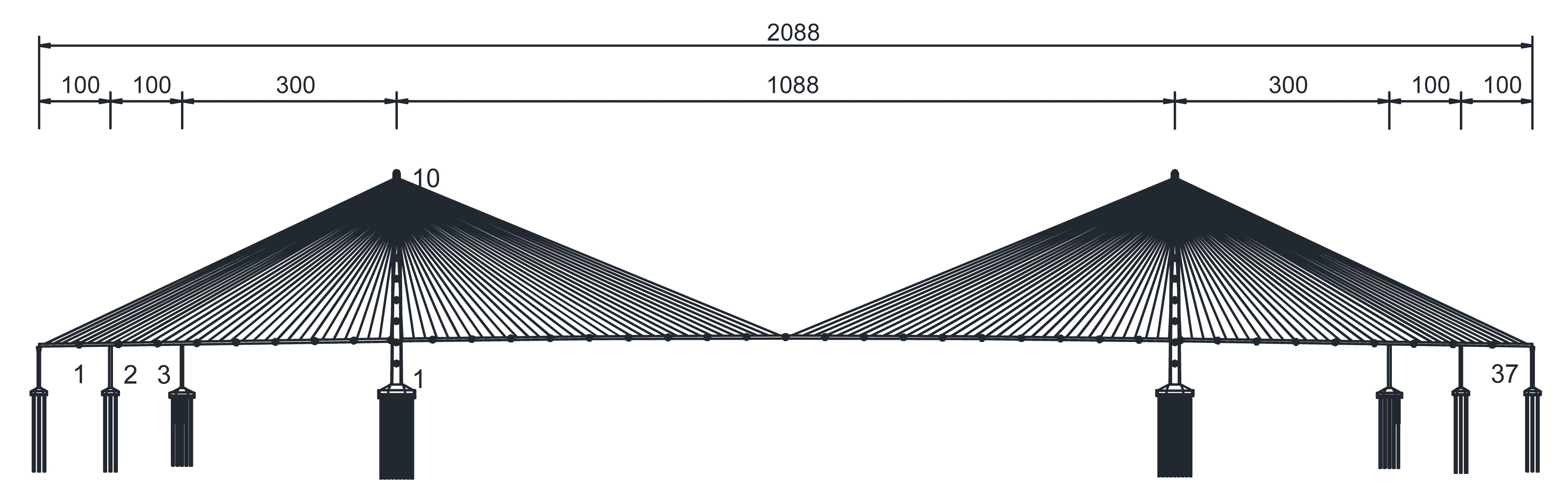Fig.4
Distribution of the simulated points for the fluctuating wind of the SCB

### 3.2.  Simulation of the fluctuating wind field for the bridge deck and towers

The spectra-representation method with fast Fourier transformation (FFT) by Deodatis in 1996 is employed to digitally simulate fluctuating wind fields for the bridge deck and towers. In the horizontal direction, the Kaimal spectrum (Kaimal, ) is adopted as shown in Eq. (1) and the Panofsky spectrum (Panofsky and McCormick, ) is employed in the vertical direction as shown in Eq. (2). Note that the parameters in the spectra are obtained from the long-term wind monitoring on the SCB (Wang et al., ). , , where Su is the power spectral density (PSD) along the wind direction, Sw is the PSD in the vertical direction, f is the Moning coordinates, n is the natural frequency of the wind speed, and u * is the wind friction speed. According to the Wind-resistant Design Specification for Highway Bridges in China (Ministry of Transport of the People’s Republic of China, ), f and u * can be calculated as , , where z is the altitude, z 0 is the roughness length, K can be taken as 0.4, U(z), which can be calculated as Eq. (5), is the wind speed at the height of z. , where U(Z 2) and U(Z 1) represent the wind speeds at the heights of Z 2 and Z 1, respectively, and α is a dimensionless exponent dependent upon the roughness of the terrain, taken as a measured value of 0.12 herein.

During the wind field simulation, the Davenport correlation function (Simiu and Scalan, ) is used to represent the wind speed correlation between the two different spatial points. Since buffeting responses around the intersection of the bridge tower and bridge span are generally low, the correlation between them is omitted. For a similar reason, the correlation between the two towers is neglected. Assuming that the simulated points of the bridge span are in the same elevation, the correlation between each point for the bridge span is only the function of the horizontal coordinates.

Considering 100 years’ of recurrence intervals, the average wind speed of the SCB site at the height of 75.6 m is set as 38.0 m/s based on the above-mentioned Specification and the long-term recorded wind data (Wang et al., ). Using the harmonic synthetic method (Deodatis, ), the fluctuating wind fields for the bridge deck and towers are simulated. Some of the fluctuating wind samples are shown in Fig. 5.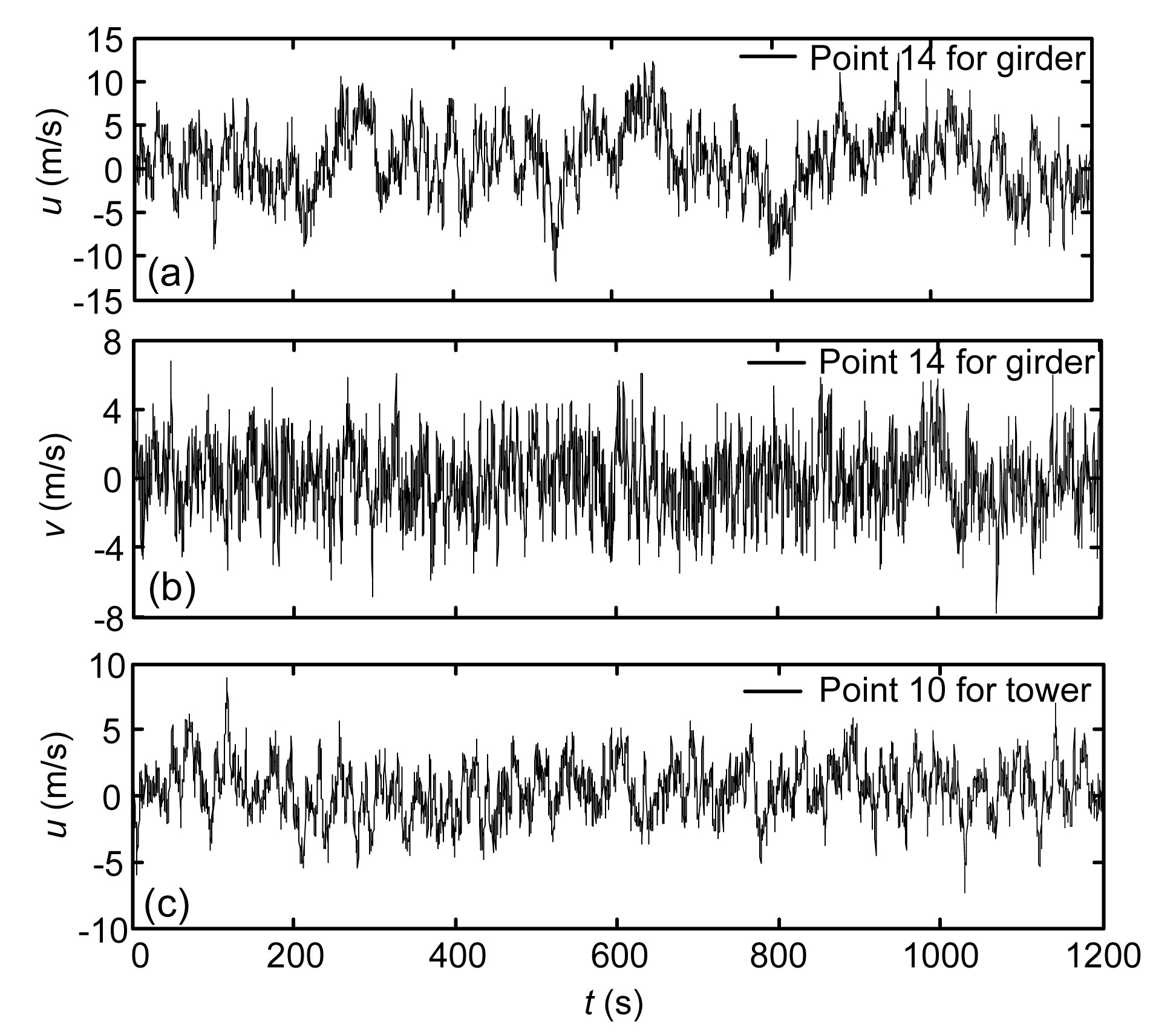Fig.5
Samples of the fluctuating wind field at the SCB site
(a) Point 14 for girder along wind direction; (b) Point 14 for girder in vertical direction; (c) Point 10 for tower along wind direction

To verify the effectiveness of the simulated fluctuating wind samples, the PSDs, auto-correlation functions, and cross-correlation functions, which are calculated respectively, are taken to be comparisons with the theoretical targets.

Typically, the comparisons of the simulated and target spectra at Point 14 in both horizontal and vertical directions are shown in Fig. 6. The auto-correlation function of the generated fluctuating wind samples at Point 14 shown in Fig. 7a is plotted versus the target auto-correlation function, and the cross-correlation functions of Point 1 and Point 14 from the generated fluctuating wind samples versus the corresponding target are shown in Fig 7b. Fig. 6 and Fig. 7 show that the simulated horizontal and vertical fluctuating wind spectra can fit well with the target spectra.Fig.6
Comparison of PSDs between simulated spectra and target spectra: (a) horizontal; (b) verticalFig.7
Auto-/Cross-correlation functions of generated fluctuating wind samples versus corresponding targets
(a) R14, 14; (b) R1, 14

## 4.  Structural finite element model and dynamic properties

A 3D dynamic finite element model has been established for the SCB based on ANSYS (Wang et al., ). The spatial beam elements are used to model the girder, transverse diaphragms, towers, and piers. The stayed-cables are modeled by spatial truss element accounting for the unidirectional tensile property. Considering the nonlinear stiffness existing in the stayed-cables due to gravity, the approximate linearized stiffness is adopted using the Ernst equation of equivalent modulus of elasticity (Ernst, ). According to the practical structural patterns in the design, the rotational degree of freedoms (DOFs) of the girder and the towers are coupled in longitudinal and transverse directions; the translational DOFs of the transverse diaphragms and piers are coupled in the vertical and transverse directions. Piers that are fixed to the foundation and the soil-structure interactions are not considered.

As one type of flexible structures, the cable-stayed bridge exhibits prominent geometric nonlinearity. To acquire the natural frequencies and corresponding mode shapes, a prestressed modal analysis is performed after a large deflection static analysis. The first 200 modes of the SCB are extracted by using the subspace method, and Table 1 lists the first 20 natural frequencies and the corresponding mode shapes.

#### Table 1

The first 20 natural frequencies and mode shapes of the SCB
 No. Natural frequency (Hz) Mode direction Characteristics of modes 1 0.0637 LG Longitudinal floating vibration of the deck 2 0.1007 L The 1st symmetric lateral bending vibration 3 0.1878 V The 1st symmetric vertical bending vibration 4 0.2312 V The 1st antisymmetric vertical bending vibration 5 0.3028 L The 1st antisymmetric lateral bending vibration 6 0.3354 V The 2nd symmetric vertical bending vibration 7 0.3915 L The 2nd symmetric lateral bending vibration 8 0.3959 L The 1st symmetric lateral bending vibration of the main tower 9 0.4016 V The 1st antisymmetric lateral bending vibration of the main tower 10 0.4439 V The 3rd symmetric vertical bending vibration 11 0.4980 V The 3rd antisymmetric vertical bending vibration 12 0.5297 L+T The 2nd symmetric lateral bending vibration accompanied by torsion 13 0.5404 V The 4th symmetric vertical bending vibration 14 0.5599 V The 5th symmetric vertical bending vibration 15 0.5812 V The 4th antisymmetric vertical bending vibration 16 0.5972 L The 2nd antisymmetric lateral vibration 17 0.6290 L+T The 3rd symmetric lateral bending vibration accompanied by torsion 18 0.6423 V The 6th symmetric vertical bending vibration 19 0.6527 V The 5th antisymmetric vertical bending vibration 20 0.6783 V The 2nd antisymmetric lateral bending vibration of the main tower

• Note: LG: longitudinal; L: lateral; V: vertical; T: torsional

•  As shown in Table 1, the lowest frequency of the SCB is 0.0637 Hz, corresponding to the longitudinal floating vibration of the deck. The first lateral mode is the symmetric bending vibration of the mid-span at a frequency of 0.1007 Hz. The first vertical mode is the symmetric bending vibration of the main span at a frequency of 0.1878 Hz. The first torsional mode occurs at a frequency of 0.5297 Hz, and the second torsional mode vibrates at a frequency of 0.6290 Hz.

## 5.  Time-domain buffeting analysis of the SCB

### 5.1.  Buffeting analysis method

A quasi-steady time-domain buffeting analysis method is utilized to analyze the responses of the SCB under fluctuating winds with the self-excited aerodynamic forces included (Scanlan and Lin, ). The Quasi-steady buffeting model by Davenport is applied to consider the buffeting forces acting on the SCB. The aerodynamic admittance function is omitted because it is not available from the wind-tunnel testing. The flutter derivatives of the SCB were measured by wind-tunnel tests at Tongji University (Chen et al., ), and the self-excited aerodynamic forces acting on the bridge deck are modeled with the element Matrix 27 in ANSYS (Hua et al., ). The other procedures used to analyze structural buffeting responses have been illustrated by Wang et al. ().

### 5.2.  Buffeting responses of the SCB

Following a nonlinear static analysis of the SCB under the actions of both static wind loads and gravity, the nonlinear time-domain buffeting analysis is conducted with fluctuating wind loads and self-excited aerodynamic forces included. Among all the parts of the deck, the wind-induced buffeting responses in the middle of the main girder are the most prominent. Table 2 lists the root-mean-square (RMS) and amplitude of the output displacements’ time-histories in the middle of the main girder.

#### Table 2

Displacement responses in the middle of the main girder
 Direction RMS (mm) Amplitude (mm) Lateral 776.9 2555.0 Vertical 436.3 1371.0 Torsional 10.5 33.8

• Note: To unify the unit, the torsional displacement angle has been multiplied by a half of the deck width

•  As shown in Table 2, both the RMS and amplitude of the lateral and vertical displacements are much more remarkable than those of the torsional displacements. Therefore, the lateral and vertical buffeting responses of the SCB are mainly focused on in this study. Fig. 8 shows the time-histories of the lateral and vertical buffeting displacements in the middle of the deck. The buffeting displacement time-histories are then transformed into frequency-domain signals using the FFT technique, and the corresponding PSDs of the time-histories are shown in Fig. 9.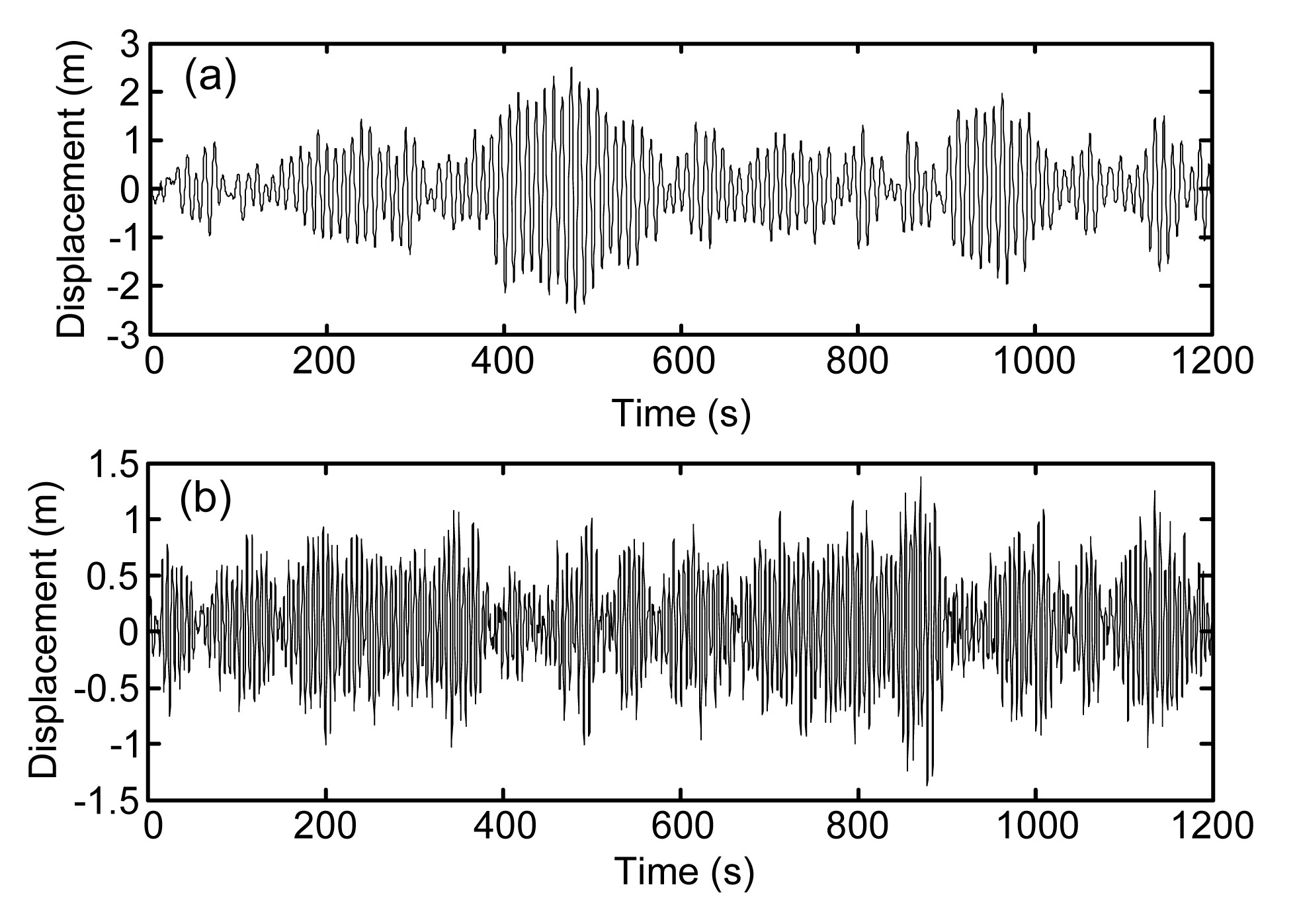Fig.8
Time-history of buffeting displacements in the middle of the bridge deck: (a) lateral; (b) vertical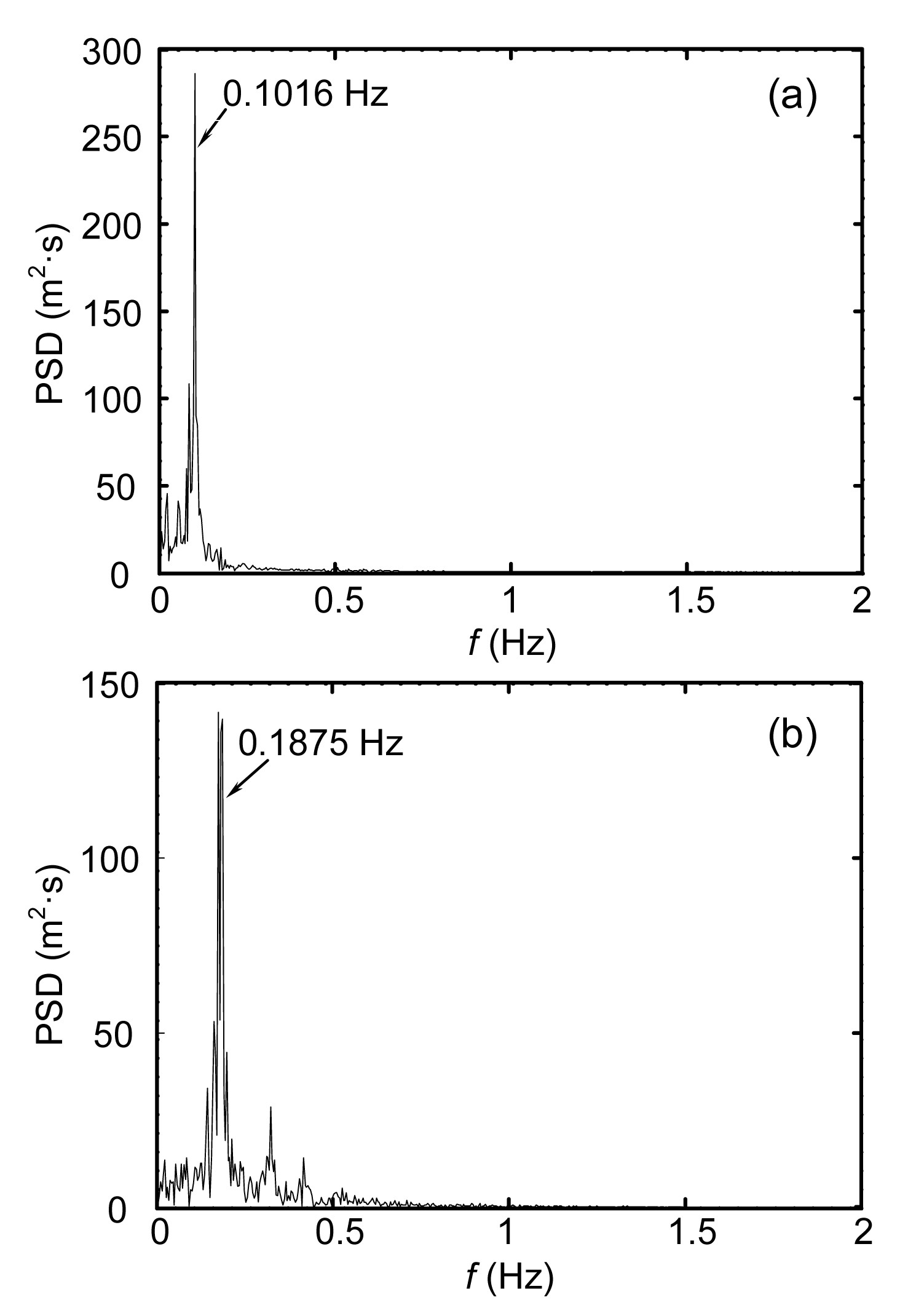Fig.9
PSDs of buffeting displacements in the middle of the bridge deck: (a) lateral; (b) vertical

As shown in Fig. 9, the energy of the lateral vibrations of the bridge deck are concentrated on 0.1016 Hz, which is close to the second-order frequency of the SCB; and the energy of the vertical vibrations is centered on 0.1875 Hz, which is close to the third-order frequency. Results indicate that the lateral buffeting responses of the SCB are dominated by the first symmetric lateral bending mode, and the vertical buffeting responses of the SCB are dominated by the first symmetric vertical bending mode. Other modes make little contribution to the buffeting responses. Thus, the buffeting control analysis afterwards will be smoothly aimed at the two influential modes.

## 6.  Application of MTMD in buffeting control of the SCB

Considering that the maximum wind-induced displacement response occurs in the middle of the bridge span, the displacement response at this section is chosen for analysis. Since the lateral and vertical buffeting responses of the SCB are dominated by the second-order and the third-order modes, respectively, the first symmetric lateral bending mode and the first symmetric vertical bending mode are determined as the controlled modes, which will be utilized for MTMD design.

### 6.1.  Layout of the MTMDs on bridge span

For structures whose dynamic responses are dominated by one structural mode, MTMDs should be deposited near the amplitude of the corresponding mode shape. So the MTMD system for the SCB is attached to the center region of the bridge girder, where the displacements of the controlled modes are the largest. The TMDs are symmetric about the central line of the main girder as well as the axial line along the bridge span. To ensure the symmetry of the TMDs installed on the span of the SCB, the number of TMDs installed on each side, to decrease vertical or lateral buffeting responses, should be odd, and then the total number of TMDs will be even. When installing the TMDs, the TMDs in the inner side are used to suppress lateral buffeting responses and those in the outer side are utilized to restrain vertical responses. Thus, there are two MTMD systems in the SCB, including the lateral MTMD system and the vertical MTMD system. The reason for this distributed arrangement of the TMDs is to avoid the phenomenon of stress concentration, so that the requirements of TMD design and installation can be satisfied. In addition, the distributed arrangement of the TMDs can control the torsional vibration of the steel box girder to some extent. Both of the two MTMD systems are installed in the steel box girder (Xing et al., ). The layout of the MTMD systems in the girder of the SCB is shown in Fig. 10.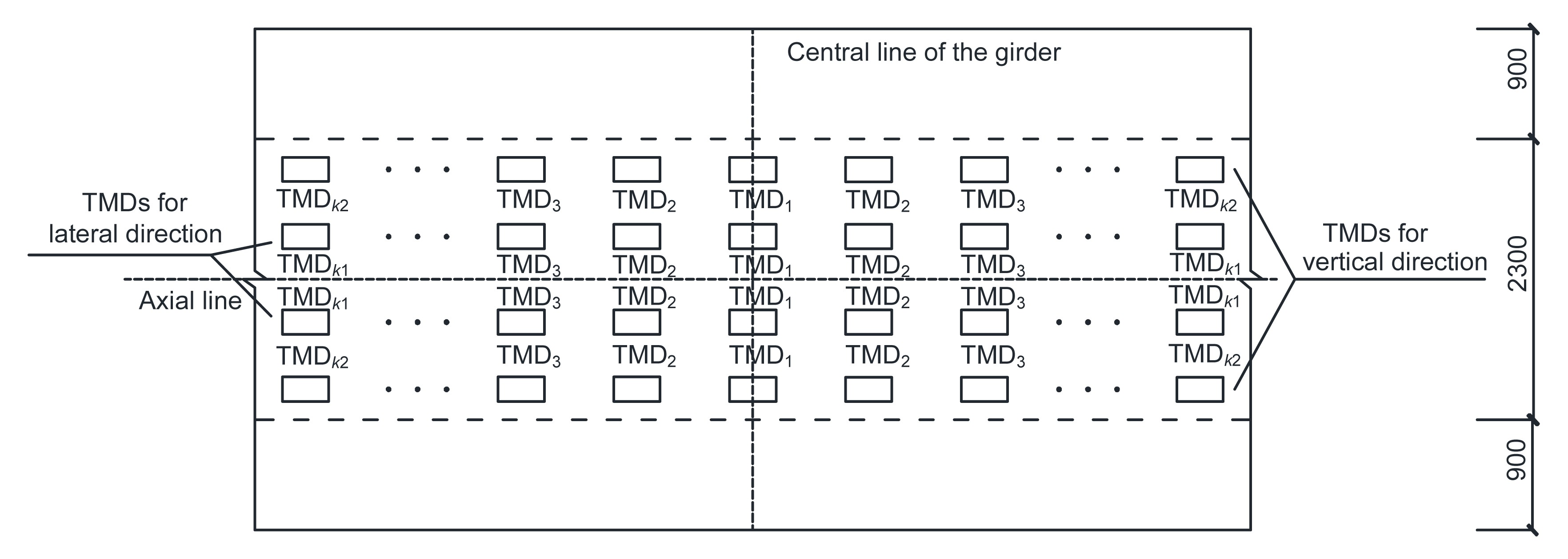Fig.10
Layout of MTMDs in the bridge girder (unit: cm)

### 6.2.  Design parameters for MTMD

The design parameters of a MTMD mainly contain the number of TMDs, the damping ratio, the mass ratio, the frequency band-width ratio, the central frequency, the central frequency ratio, etc. Since the damping ratio of the TMDs has less significant effects on the control efficiency (Igusa and Xu, ), the damping ratios for all the TMDs are assumed to be the same as ζ in this study. The mass ratio refers to the ratio between the total mass of TMDs in a MTMD and the equivalent mass of the controlled mode, which is defined as , where mi is the mass of a TMD, N means the number of TMDs, and M is the equivalent mass of the controlled mode.

Assuming that the lowest frequency belongs to the central TMD, namely TMD1 in Fig. 10, then the frequencies of the other TMDs increase with an equal frequency increment towards both ends of the MTMD system. As an average of the TMDs’ frequencies in either side of the MTMD, the central frequency can be defined as , where k=(N+2)/4, and fi is the frequency of TMD i .

The central frequency ratio is the ratio between the central frequency and the controlled frequency of the structure, and it is set as 1.0. The frequency band-width ratio is the ratio between the frequency band-width and the controlled frequency of the structure, which is defined as , where f 1 and fk are the frequencies of TMD1 and TMD k , respectively; and f s is the natural frequency of the controlled mode.

To reflect the control level of the MTMD on the SCB, a formula is proposed to quantify the control efficiency as , where α and β are the buffeting displacement RMS values in the middle of the bridge deck with and without MTMD, respectively.

### 6.3.  Finite element modeling of the MTMD

MTMD is composed of a series of distributed TMDs, thus the modeling of the MTMD can be transferred to the simulation of several TMDs. Since there is no element of TMD included in ANSYS, the simulation of a TMD herein takes on the advantages of Mass21 and Combin14 (Gu et al., ; Nguyen et al., ). The mass blocks of the TMDs are simplified into individual masses by the element Mass21 in ANSYS. Mass21 is a single-node element with six DOFs: translations in the nodal x, y, and z directions, and rotations about the nodal x, y, and z axes. A different mass and rotary inertia may be assigned to each coordinate direction. The features of Mass21 are shown in Fig. 11a. The spring and the damper of a TMD are simulated by the element Combin14. The element is a uniaxial tension-compression element which has three DOFs for each node, as shown in Fig. 11b. The spring constant and the damping coefficient reflect the functions of the spring and the damper in a TMD.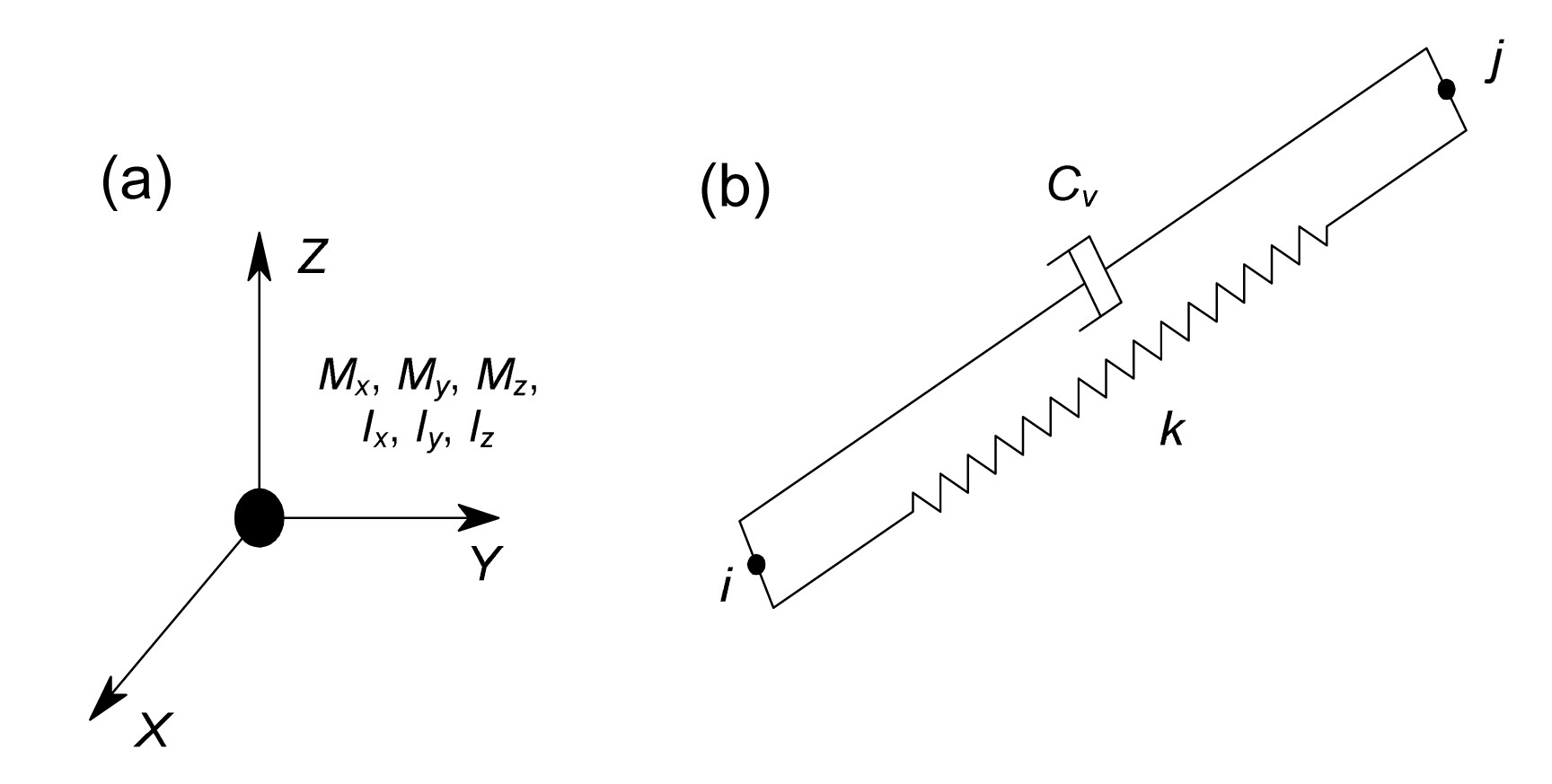Fig.11
Features of Mass21 element (a) and Combin14 element (b)

### 6.4.1.  The number of TMDs

When only a small number of TMDs are utilized, the MTMD might not be robust enough to handle the frequency variation due to the wind and vehicles’ excitation (Gu et al., ). But if the number of TMDs exceeds a critical level, some other problems such as high cost and inconvenience will take place. Thus, a proper number of TMDs should be determined in the MTMD. Fig. 12 shows the control efficiency of the MTMD versus the number of TMDs. The mass ratio, the frequency band-width ratio, and the damping ratio are set as 0.01, 0.1, and 0.02, respectively. In both the lateral and the vertical MTMDs, the layout of the TMDs is symmetric about the central line of the bridge girder. During the analysis, the increments of the TMDs in both lateral and vertical MTMD systems are set as four.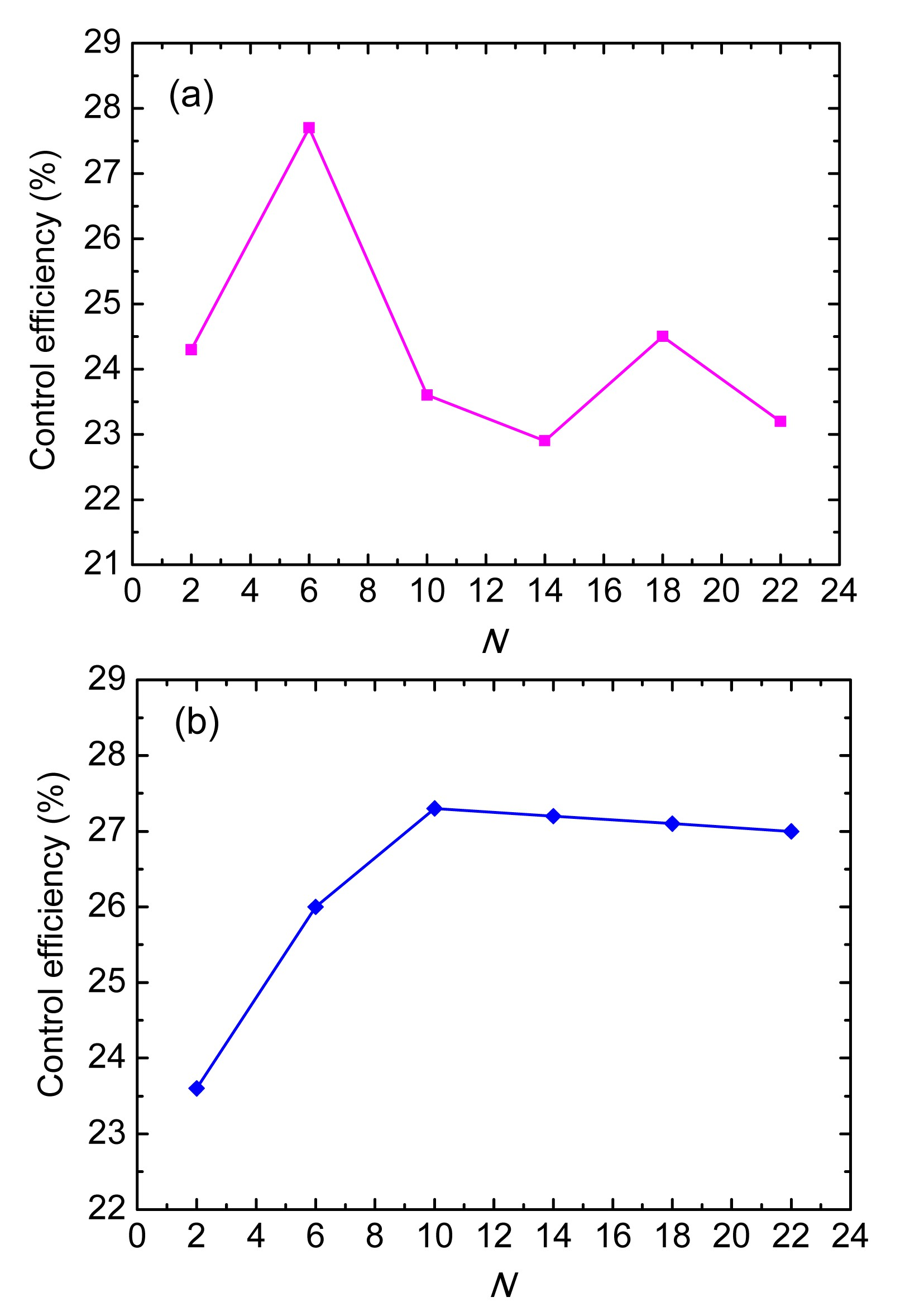Fig.12
Influence of the number of TMDs on the control efficiency: (a) lateral; (b) vertical

As shown in Fig. 12, in general, the control efficiency of the MTMD is sensitive to the number of TMDs. Specifically, the overall influence trend of the number of TMDs on the control efficiency of the lateral buffeting responses is fluctuant and the control efficiency reaches the peak with six TMDs. The control efficiency gets its second peak value when the number of TMDs is 18. With regard to the vertical buffeting, the control efficiency increases with the increment of the number of TMDs when the number is less than 10, and the rate of rise is relatively large. The control efficiency decreases slowly after it reaches a peak with 10 TMDs. In addition, the frequency distribution of the MTMD system will be dispersive with a small number of TMDs at a constant frequency band-width ratio, which is not favorable for handling the frequency variation of the SCB. As a result, the number of TMDs in a MTMD should be determined with the control efficiency and robustness considered comprehensively.

### 6.4.2.  Mass ratio

The mass ratio is another vital parameter of MTMD. In this procedure, the frequency band-width ratio is set as 0.1, the damping ratio is taken as 0.02, and the number of TMDs is set as 18 for the lateral direction and 10 for the vertical direction. The control efficiencies of the lateral and vertical buffeting responses versus the mass ratio can be seen in Fig. 13.Fig.13
Influence of the mass ratio on the control efficiency: (a) lateral; (b) vertical

As shown in Fig. 13, for both lateral and vertical buffeting displacements, the control efficiency increases with the increment of the mass ratio. It seems that a larger TMD’s mass would give a better control performance, but the total mass was limited to the available budget and construction convenience. Thus, a proper mass ratio of the MTMD system should consider these factors including the control efficiency, the available budget, and the construction convenience.

### 6.4.3.  Frequency band-width ratio

Taking account of 18 TMDs for the lateral direction and 10 TMDs for the vertical direction at a mass ratio of 1%, the influence of the frequency band-width ratio ranging from 0.05 to 0.40 on the control efficiency is analyzed with the damping ratio chosen as 0.02. Fig. 14 shows the control efficiencies of the lateral and vertical buffeting displacements versus the frequency band-width ratio.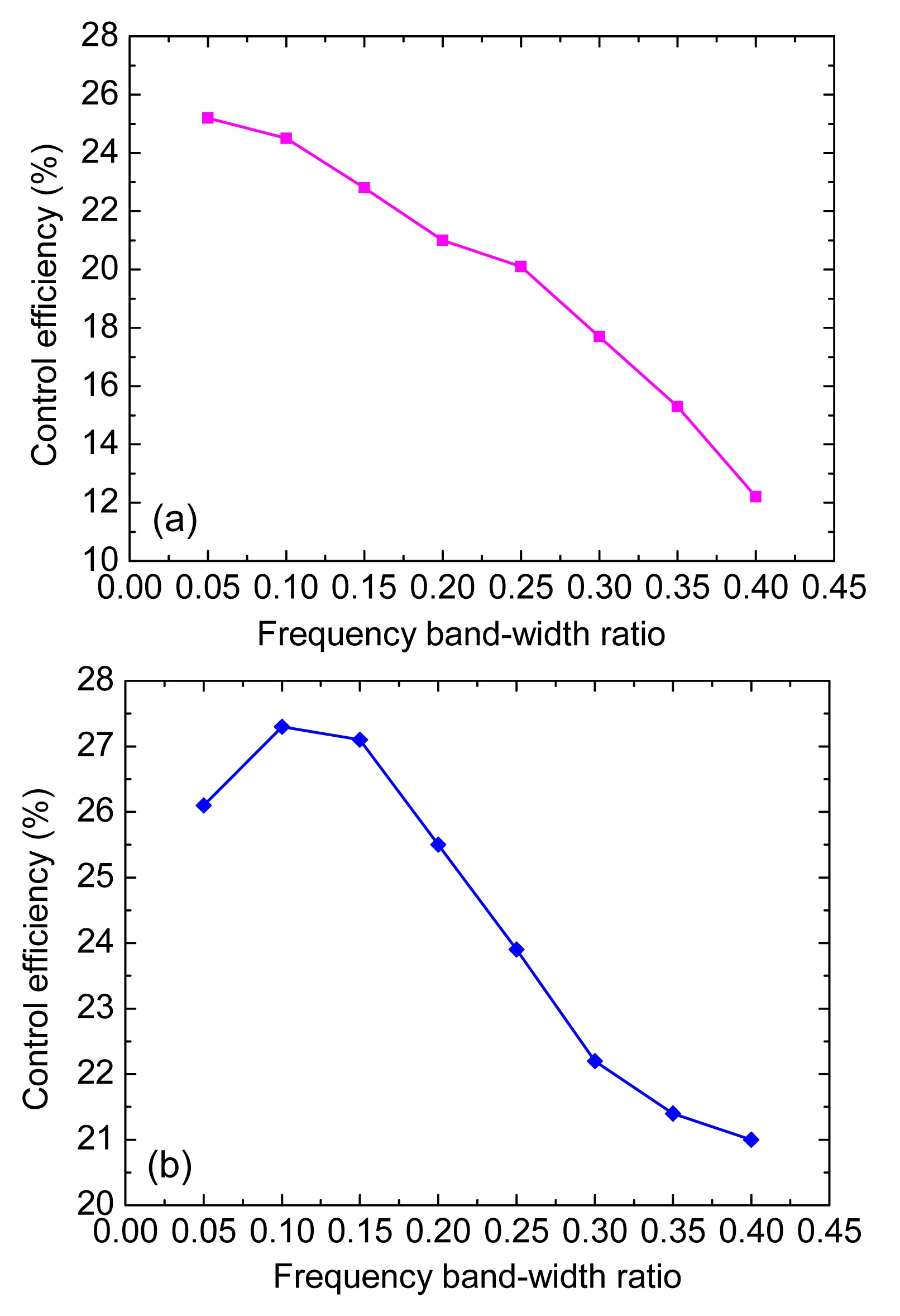Fig.14
Influence of the frequency band-width ratio on the control efficiency: (a) lateral; (b) vertical

As shown in Fig. 14, the control efficiency of the lateral displacements decreases with the increment of the frequency band-width ratio, while the control efficiency of the vertical displacements increases before the frequency band-width ratio reaches 0.10 and then decreases. A smaller frequency band-width ratio leads to higher control efficiencies in the lateral direction, but it will lead to a narrow frequency band-width which is bad for the robustness of the MTMD. Therefore, the determination of the frequency band-width ratio should take both the control efficiency and the robustness of a MTMD system into account.

### 6.4.4.  Damping ratio

During the analysis, the damping ratio of all TMDs in a MTMD system is assumed to be the same. The control efficiencies of the lateral and vertical buffeting displacements versus the damping ratio are analyzed while other parameters are kept constant (the number of TMDs: 18 for lateral directions, 10 for vertical directions; mass ratio: 1%; frequency band-width ratio: 0.1), as shown in Fig. 15. Fig. 15 shows that the control efficiencies of the lateral and vertical buffeting displacements increase with the rise of the damping ratio to peak values and decrease afterwards. The damping ratio of the TMD in a MTMD system is sensitive to the control efficiencies of the buffeting displacements.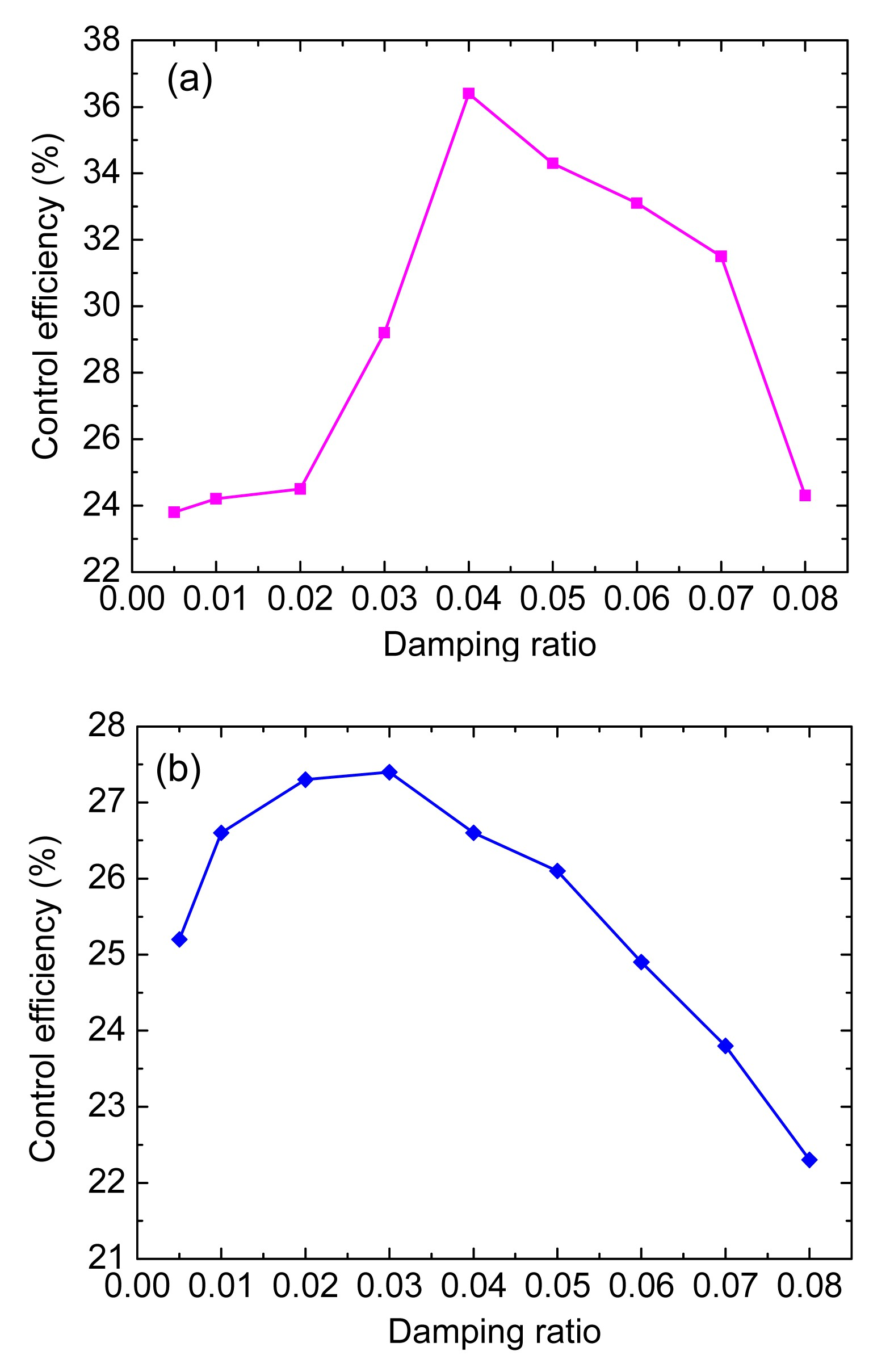Fig.15
Influences of the damping ratio on the control efficiency: (a) lateral; (b) vertical

### 6.5.  Optimal parameters’ determination of the MTMD system

The sensitivity analysis reveals the sensitive characteristics of the control efficiency to the design parameters of MTMD, and the selection of the design parameters is closely related to the overall costs, construction difficulty, and robustness of a MTMD system. Hence, it is essential to search for an optimal combination of the design parameters to balance all the target factors. In order to realize the optimization in this analysis, the selection of design parameters is transformed into a multi-objective nonlinear optimization problem under constraints.

There are several techniques available to solve the constrained optimization problem (Agrawal and Yang, ; Wang et al., ). Among them, the first-order optimization method is utilized to determine the optimal parameters of the MTMD of the SCB. Considering the control efficiency, cost, construction difficulty, and robustness of a MTMD system, an objective function J evaluating all the target factors is proposed. Then the optimization problem can be formed as

Minimize , where E(μ, ζ) is the material cost function correlated to the mass ratio μ and the damping ratio ζ, E(μ,ζ)=μζ 2; C(N) is the construction difficulty function related to the number of TMDs, C(N)=2N; F(δ) is the control efficiency evaluating function of the MTMD system, F(δ)=δ 2; and R(N, η) is the robustness evaluating function of the MTMD system, R(N, η)=ηN 1/2.

The above procedure is carried out by using the optimization module (/OPT) in ANSYS and the first-order optimization method is applied to obtain the optimal parameters of the MTMD system (Wang et al., ). The lower and upper bounds of the design parameters of the MTMD are determined according to the above sensitivity analysis results and engineering experience (Table 3).

#### Table 3

The lower and upper bounds of the design parameters of the MTMD
 Direction Number of TMDs Mass ratio Damping ratio Frequency band-width ratio Lateral [2.0, 22.0] [0.5%, 2.0%] [0.03, 0.07] [0.05, 0.25] Vertical [2.0, 22.0] [0.5%, 1.5%] [0.01, 0.05] [0.05, 0.25]

To avoid the local minimum, the zero-order optimization method is employed to validate the optimization results in this study. The optimal parameters of the MTMD system which can satisfy the target control efficiency and other qualifications are finally determined (Table 4).

#### Table 4

General optimal parameters for the MTMD system on the SCB
 Direction Number of TMDs Mass ratio Damping ratio Frequency band-width ratio Lateral 18 1% 0.04 0.10 Vertical 10 1% 0.03 0.10

### 6.6.  Comparative study on buffeting responses with and without MTMD

The detailed optimal parameters for the MTMD are shown in Table 5, and the corresponding lateral and vertical buffeting displacements are shown in Fig. 16.

#### Table 5

Detailed optimal parameters for the MTMD systems on the SCB
 Direction SNo. No. M (t) K (kN/m) C (t/s) Lateral 1 2 8.8 3.182 0.423 2 4 8.8 3.350 0.434 3 4 8.8 3.523 0.445 4 4 8.8 3.700 0.456 5 4 8.8 3.881 0.468 μ=1%; γ=0.10; ζ=0.04 Vertical 1 2 10 12.57 0.673 2 4 10 13.92 0.708 3 4 10 15.35 0.743 μ=1%; γ=0.10; ζ=0.03

• Note: SNo. is the serial number of TMDs as shown in Fig. 10; No. is the number of TMDs in each group; M is the mass for each TMD; K is the stiffness coefficient; C is the damping coefficient; μ is the mass ratio of the MTMD system; γ is the frequency band-width ratio; and ζ is the damping ratio for each TMD

•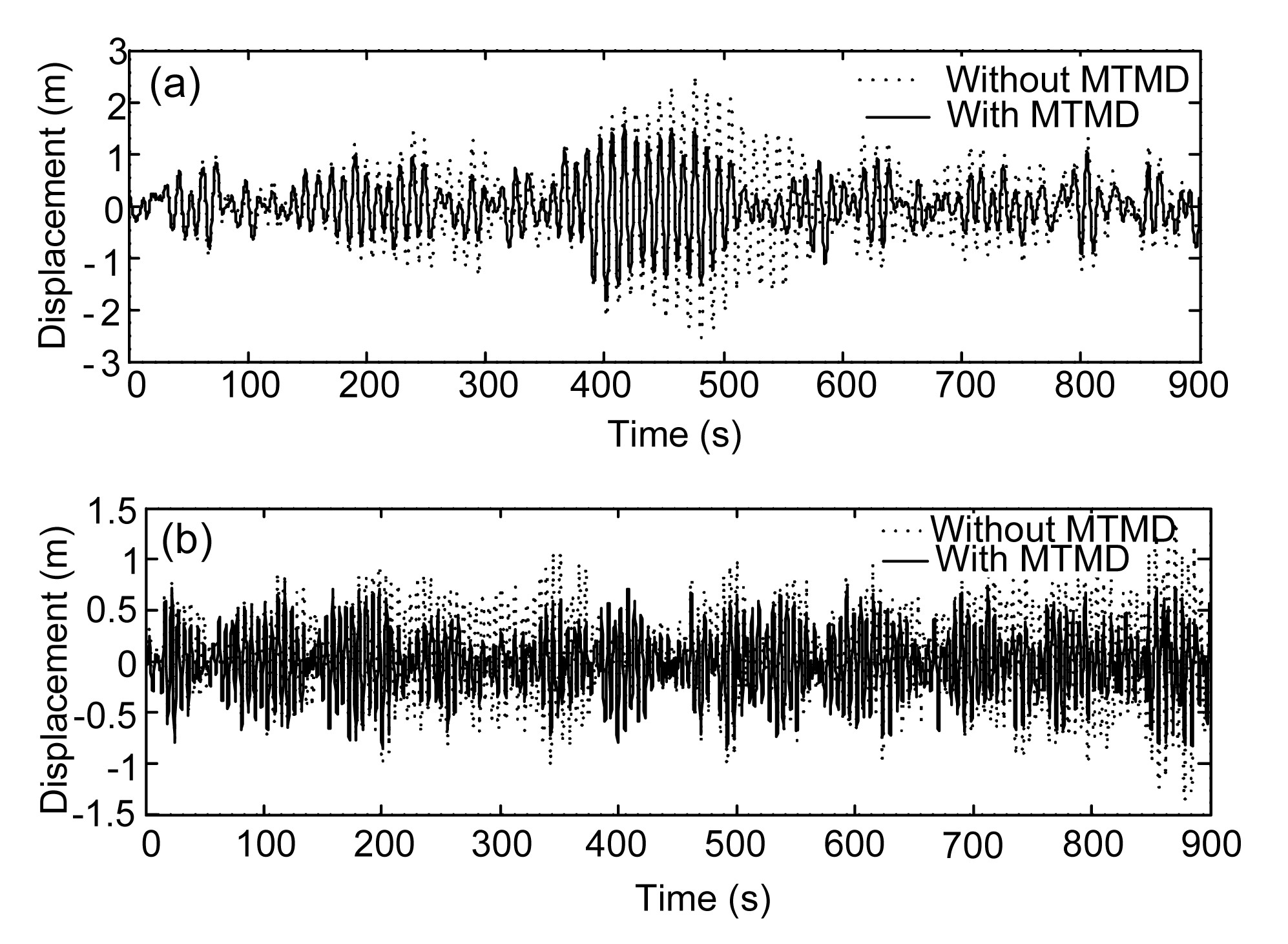Fig.16
Buffeting displacements of the SCB with and without MTMD: (a) lateral; (b) vertical

Fig. 16 shows that the control efficiencies of the lateral and vertical buffeting displacements on the SCB can reach 36.4% and 27.4%, respectively. The MTMD can effectively suppress the buffeting displacement of the SCB, and the control effect in the lateral direction is better than that in the vertical direction. The PSDs of the lateral and vertical displacements are shown in Fig. 17.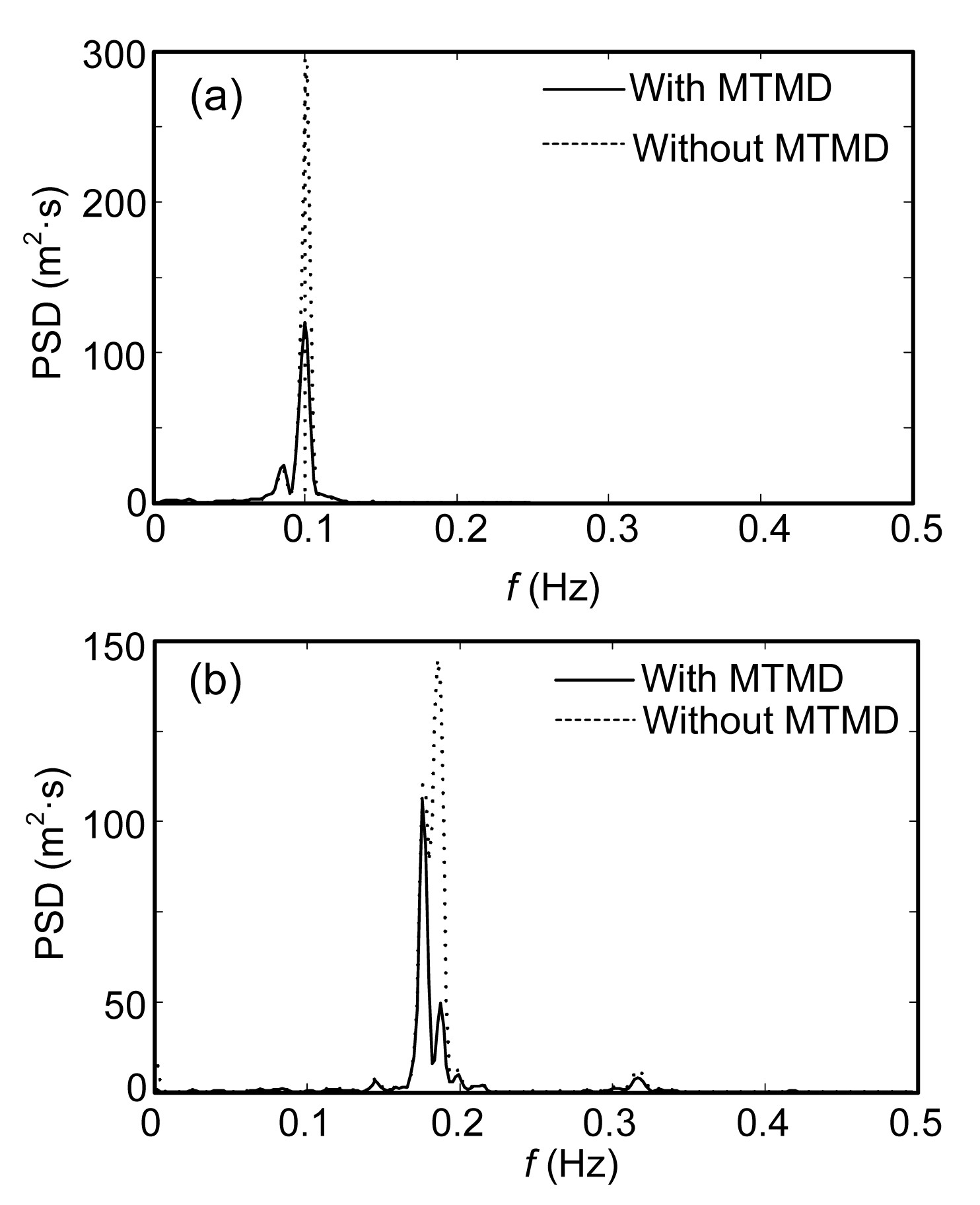Fig.17
PSDs of the buffeting displacements of the SCB with and without MTMD: (a) lateral; (b) vertical

In the frequency domain, it is obvious that the energy of the structural vibrations can be effectively suppressed by MTMD, which is consistent with the results in the time-domain. The lateral MTMD performs a better control effect than the vertical MTMD. The prominent control effect of the MTMD can also indirectly verify the contributions of the first-order lateral bending mode and the first-order vertical bending mode to the wind-induced buffeting responses. In conclusion, the MTMD with reasonable mechanical parameters can effectively suppress wind-induced buffeting responses of the SCB.

## 7.  Conclusions

The following conclusions can be drawn from the study on the optimal control of the buffeting displacement of the SCB with MTMD.

1. The PSDs of the buffeting displacements show that the first-order lateral bending mode dominates the lateral wind-induced buffeting responses of the SCB, and the first-order vertical bending mode dominates the vertical wind-induced buffeting responses.

2. The control efficiency is sensitive to the parameters of MTMD including the number of TMD, mass ratio, frequency band-width ratio, and damping ratio. The mass ratio and frequency band-width ratio are relatively sensitive among these influence parameters.

3. For wind-induced buffeting displacements in both the lateral and vertical directions, the control efficiency increases positively with the increment of the mass ratio. However, the total mass of the MTMD is limited to the economic and construction factors.

4. The control efficiency of the lateral displacements decreases with the increment of the frequency band-width ratio, while the control efficiency of the vertical displacements increases before the frequency band-width ratio reaches 0.10 and decreases when the ratio becomes larger than 0.10.

5. By using the optimization module in ANSYS, the optimal parameters of the MTMD system on the SCB can be conveniently obtained based on the first-order optimization method, and the optimal results can then be verified by the zero-order optimization method.

6. Both the vertical and the lateral vibrations can be effectively controlled when the optimal design parameters of MTMD system are used, and the lateral MTMD system performs a better control effect than the vertical MTMD system, which has been proved in both time-domain and frequency-domain. Therefore, MTMD systems with optimal mechanical parameters are thought to be an efficient method to suppress buffeting responses of the SCB.

* Project supported by the Major State Basic Research Development Program of China (973 Program) (No. 2015CB060000), the National Natural Science Foundation of China (Nos. 51378111 and 51438002), the Fok Ying-Tong Education Foundation for Young Teachers in the Higher Education Institutions of China (No. 142007), the Program for New Century Excellent Talents in University of Ministry of Education of China (No. NCET-13-0128), and the Jiangsu Key Laboratory of Environmental Impact and Structural Safety in Engineering, China University of Mining & Technology (No. JSKL2014K03), China

## ReferencesAbe, M., Fujino, Y., 1994. Dynamic characterization of multiple tuned mass dampers and some design formulas. Earthquake Engineering and Structural Dynamics, 23(8):813-835.Agrawal, A.K., Yang, J.N., 2000. Optimal placement of passive dampers on seismic and wind-excited buildings using combinatorial optimization. Journal of Intelligent Material Systems and Structures, 10(12):997-1014.Chen, A.R., You, Q.Z., Zhang, X.G., 2005. Aerodynamic problems of a super-long span cable-stayed bridge. , Proceedings IABSE Symposium, International Association for Bridge and Structural Engineering, Zurich, Switzerland, 74-81. :74-81.Chen, X.Z., Kareem, A., 2002. Advanced analysis of coupled buffeting response of bridges: a complex modal decomposition approach. Probabilistic Engineering Mechanics, 17(2):201-213.Chen, Z.Q., Han, Y., Hua, X.G., 2009. Investigation on influence factors of buffeting response of bridges and its aeroelastic model verification for Xiaoguan Bridges. Engineering Structures, 31(2):417-431.Chan, P.W., Lee, Y.F., 2013. Performance of LIDAR- and radar-based turbulence intensity measurement in comparison with anemometer-based turbulence intensity estimation based on aircraft data for a typical case of terrain-induced turbulence in association with a typhoon. Journal of Zhejiang University-SCIENCE A (Applied Physics & Engineering), 14(7):469-481.Den Hartog, J.P., 1956.  Mechanical Vibration. McGraw-Hill,New York :Deodatis, G., 1996. Simulation of ergodic multivariate stochastic processes. Journal of Engineering Mechanics, 122(8):778-787.Ernst, J.H., 1965. Der e-modul von seilen unter berucksichtigung des durchhanges. Der Bauingenieur, (in German),40(2):52-55.Fujino, Y., Abe, M., 1993. Design formulas for tuned mass dampers based on a perturbation technique. Earthquake Engineering and Structural Dynamics, 22(10):833-854.Foster, S., Chan, P.W., 2012. Improving the wind and temperature measurements of an airborne meteorological measuring system. Journal of Zhejiang University-SCIENCE A (Applied Physics & Engineering), 13(10):723-746.Gu, M., Chen, S.R., Chang, C.C., 2001. Parametric study on multiple tuned mass dampers for buffeting control of Yangpu Bridge. Journal of Wind Engineering and Industrial Aerodynamics, 89(11-12):987-1000.Hoang, N., Fujino, Y., Warnitchai, P., 2008. Optimal tuned mass damper for seismic applications and practical design formulas. Engineering Structures, 30(3):707-715.Hua, X.G., Chen, Z.Q., Ni, Y.Q., 2007. Flutter analysis of long-span bridges using ANSYS. Wind and Structures, 10(1):61-82.Igusa, T., Xu, K., 1994. Vibration control using multiple tuned dampers. Journal of Sound and Vibration, 175(4):491-503.Kaimal, J.C., 1972. Spectral characteristics of surface layer turbulence. Quarterly Journal of the Royal Meteorological Society, 98(417):563-589.Kareem, A., Kline, S., 1995. Performance of multiple mass dampers under random loading. Journal of Structural Engineering, 121(2):348-361.Li, C.X., 2002. Optimum multiple tuned mass dampers for structures under the ground acceleration based on DDMF and ADMF. Earthquake Engineering and Structural Dynamics, 31(4):897-919.Marano, G., Greco, R., 2011. Optimization criteria for tuned mass dampers for structural vibration control under stochastic excitation. Journal of Vibration and Control, 17(5):679-688.Marano, G., Greco, R., Trentadue, F., 2007. Constrained reliability-based optimization of linear tuned mass dampers for seismic control. International Journal of Solids and Structures, 44(22-23):7370-7388.Marano, G., Greco, R., Chiaia, B., 2010. A comparison between different optimization criteria for tuned mass dampers design. Journal of Sound and Vibration, 329(23):4880-4890.Ministry of Transport of the Peoples Republic of China, 2004.  Wind Resistant Design Specification for Highway Bridges. Standards Press of China,Beijing :Nguyen, T.H., Saidi, I., Gad, E.F., 2012. Performance of distributed multiple viscoelastic tuned mass dampers for floor vibration applications. Advances in Structural Engineering, 15(3):547-562.Panofsky, H.A., McCormick, R.A., 1960. The spectrum of vertical velocity near the surface. Journal of the Royal Metaorological Society, 86(370):546-564.Rdinger, F., 2006. Optimal vibration absorber with nonlinear viscous power law damping and white noise excitation. Journal of Engineering Mechanics, ASCE, 132(1):46-53.Scanlan, R.H., Lin, W.H., 1978. Effects of turbulence on bridge flutter derivatives. Journal of the Engineering Mechanics Division, ASCE, 104(4):719-733.Simiu, E., Scanlan, R.H., 1996.  Wind Effects on Structures, 3rd Edition. John Wiley & Sons, Inc.,New York :Swanson Analysis Systems, 2004. ANSYS Users Manual, Version 8.0, Hoston :Wang, H., Li, A.Q., Jiao, C.K., 2010. Damper placement for seismic control of super-long-span suspension bridges based on the first-order optimization method. Science China Technological Sciences, 53(7):2008-2014.Wang, H., Li, A.Q., Hu, R.M., 2011. Comparison of ambient vibration response of the Runyang Suspension Bridge under skew winds with time-domain numerical predictions. Journal of Bridge Engineering, 16(4):513-526.Wang, H., Zong, Z.H., Li, A.Q., 2012. Digital simulation of 3D turbulence wind field of Sutong Bridge based on measured wind spectra. Journal of Zhejiang University-SCIENCE A (Applied Physics & Engineering), 13(2):91-104.Wang, H., Hu, R.M., Xie, J., 2013. Comparative study on buffeting performance of Sutong Bridge based on design and measured spectrum. Journal of Bridge Engineering, 18(7):587-600.Wang, H., Li, A.Q., Niu, J., 2013. Long-term monitoring of wind characteristics at Sutong Bridge site. Journal of Wind Engineering and Industrial Aerodynamics, 115:39-47.Xing, C.X., Wang, H., Li, A.Q., 2014. Study on wind-induced vibration control of a long-span cable-stayed bridge using TMD-type counterweight. Journal of Bridge Engineering, 19(1):141-148.Xu, Y.L., Sun, D.K., Ko, J.M., 1998. Buffeting analysis of a long span bridges: a new algorithm. Computers and Structures, 68(4):303-313.